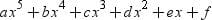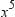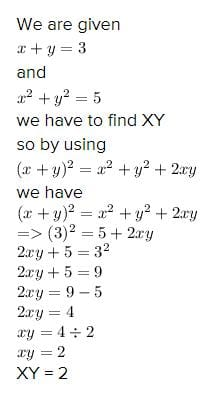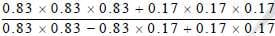Test: Polynomials - 1

# Test: Polynomials - 1

Test Description

## 25 Questions MCQ Test Mathematics (Maths) Class 9 | Test: Polynomials - 1

Test: Polynomials - 1 for Class 9 2023 is part of Mathematics (Maths) Class 9 preparation. The Test: Polynomials - 1 questions and answers have been prepared according to the Class 9 exam syllabus.The Test: Polynomials - 1 MCQs are made for Class 9 2023 Exam. Find important definitions, questions, notes, meanings, examples, exercises, MCQs and online tests for Test: Polynomials - 1 below.
Solutions of Test: Polynomials - 1 questions in English are available as part of our Mathematics (Maths) Class 9 for Class 9 & Test: Polynomials - 1 solutions in Hindi for Mathematics (Maths) Class 9 course. Download more important topics, notes, lectures and mock test series for Class 9 Exam by signing up for free. Attempt Test: Polynomials - 1 | 25 questions in 25 minutes | Mock test for Class 9 preparation | Free important questions MCQ to study Mathematics (Maths) Class 9 for Class 9 Exam | Download free PDF with solutions
 1 Crore+ students have signed up on EduRev. Have you?
Test: Polynomials - 1 - Question 1

### A cubic polynomial is a polynomial with degree

Detailed Solution for Test: Polynomials - 1 - Question 1

A cubic polynomial is a polynomial of degree 3. A univariate cubic polynomial has the form f(x) = a3 x3 + a2 x2 + a1 x + a0. An equation involving a cubic polynomial is called a cubic equation. A closed-form solution known as the cubic formula exists for the solutions of an arbitrary cubic equation.

Test: Polynomials - 1 - Question 2

### A polynomial of degree 5 in x has at most

Detailed Solution for Test: Polynomials - 1 - Question 2

A polynomial of degree 5 is of the form p(x) =, where a, b, c, d, e, and f are real numbers and a ≠ 0.

Thus, p(x) can have at most 6 terms and at least one term containingTest: Polynomials - 1 - Question 3

### The coefficient of x3 in the polynomial 5 + 2x + 3x2 – 7x3 is

Test: Polynomials - 1 - Question 4

The quadratic polynomial whose sum of zeroes is 3 and the product of zeroes is –2 is :

Detailed Solution for Test: Polynomials - 1 - Question 4

Sum of zeros = 3/1
-b/a = 3/1 .....................(1)

Product of zeros = -2/1
c/a = -2/1 ...................(2)

From equation (1) and (2)
a = 1
-b = 3, b = -3
c = -2

ax^2+by+c
= x2-3x-2

Test: Polynomials - 1 - Question 5

A linear polynomial :-

Detailed Solution for Test: Polynomials - 1 - Question 5

A number of zeroes of an n -degree polynomial = n.

First, a linear polynomial is in the form of ax + b, a≠0, a,b ∈R

The degree of the polynomial = highest degree of the terms
So here the highest degree is 1.

Hence, Linear polynomial has only one zero.

Test: Polynomials - 1 - Question 6

If x + y = 3, x2 + y2 = 5 then xy is

Detailed Solution for Test: Polynomials - 1 - Question 6Test: Polynomials - 1 - Question 7

When the polynomial x3 + 3x2 + 3x + 1 is divided by x + 1, the remainder is :-

Detailed Solution for Test: Polynomials - 1 - Question 7

The zero of x + 1 is –1

And by remainder theorem, when

p(x) = x3 + 3x2 + 3x + 1 is divided by x + 1, then remainder is p(–1).

∴ p(–1) = (–1)3 + 3 (–1)2 + 3(–1) + 1

= –1 + (3 × 1) + (–3) + 1

= –1 + 3 – 3 + 1

= 0

Thus, the required = 0

Test: Polynomials - 1 - Question 8

If the polynomial 2x3 – 3x2 + 2x – 4 is divided by x – 2, then the remainder is :-

Test: Polynomials - 1 - Question 9

The value of k for which x – 1 is a factor of the polynomial 4x3+ 3x2 – 4x + k is :-

Detailed Solution for Test: Polynomials - 1 - Question 9

X - 1 is a factor of 4x3 + 3x2 -4x +k
then x=1 is one root of 4x3 + 3x2 -4x +k

put x= 1
4x3 +3x2 -4x +k = 0

=> 4 (1)3 +3 (1)2-4 (1) +k =0

=> 4 + 3 - 4 + k = 0

=> k = -3

Test: Polynomials - 1 - Question 10

The value of k for which x + 1 is a factor of the polynomial x3 + x2 + x + k is :-

Test: Polynomials - 1 - Question 11

The value of m for which x – 2 is a factor of the polynomial x4 – x3 + 2x2 – mx + 4 is :-

Test: Polynomials - 1 - Question 12

The factors of 2x2 – 3x – 2 are :-

Detailed Solution for Test: Polynomials - 1 - Question 12

2x2 – 3x – 2

2x2 - 4x + x - 2 = 0

2x2(x-2) +1(x-2) = 0

(2x+1) (x-2)

Test: Polynomials - 1 - Question 13

If x + 2 is a factor of x3 – 2ax2 + 16, then value of a is

Test: Polynomials - 1 - Question 14

The factors of x3 – 2x2 – 13x – 10 are :-

Test: Polynomials - 1 - Question 15

The expanded form of (2x – 3y – z)2 is :-

Test: Polynomials - 1 - Question 16

The expanded form of (x + y + 2z)2 is :-

Test: Polynomials - 1 - Question 17

The expanded form of (x+1/3)3 is :-

Test: Polynomials - 1 - Question 18

x3 + y3 + z3 – 3xyz is :-

Detailed Solution for Test: Polynomials - 1 - Question 18

We know that x3 + y3 + z3 – 3xyz = (x + y + z) (x2 + y2 + z2 – xy – yz – zx).
If x + y + z = 0, then x3 + y3 + z3 – 3xyz = 0 or x3 + y3 + z3 = 3xyz.

Test: Polynomials - 1 - Question 19

(a – b)3 + (b – c)3 + (c – a)3 is equal to :-

Detailed Solution for Test: Polynomials - 1 - Question 19

Let x = (a – b), y = (b – c) and z = (c – a)
Consider, x + y + z = (a – b) + (b – c) + (c – a) = 0
⇒ x3 + y3 + z3 = 3xyz
That is (a – b)3 + (b – c)3 + (c – a)3 = 3(a – b)(b – c)(c – a)

Test: Polynomials - 1 - Question 20is equal to :-

Test: Polynomials - 1 - Question 21

√2 is a polynomial of degree

Detailed Solution for Test: Polynomials - 1 - Question 21

The highest power of the variable is known as the degree of the polynomial.

√2x^0 = √2
hence the degree of the polynomial is zero.

Test: Polynomials - 1 - Question 22

The degree of the polynomial 4x4+0x3+0x5+5x+74x4+0x3+0x5+5x+7 is

Detailed Solution for Test: Polynomials - 1 - Question 22

The degree of the polynomial 4x4+0x3+0x5+5x+74x4+0x3+0x5+5x+7 is

Test: Polynomials - 1 - Question 23

The degree of the zero polynomial is

Detailed Solution for Test: Polynomials - 1 - Question 23

The degree of zero polynomial is not defined, because, in zero polynomial, the coefficient of any variable is zero i.e., Oxor Ox5, etc. Hence, we cannot exactly determine the degree of the variable.

Test: Polynomials - 1 - Question 24

The value of the polynomial 5x−4x2+3, when x = −1 is

Detailed Solution for Test: Polynomials - 1 - Question 24

Let p (x) = 5x – 4x2 + 3 …(i)
On putting x = -1 in Eq. (i), we get
p(-1) = 5(-1) -4(-1)2 + 3= - 5 - 4 + 3 = -6

Test: Polynomials - 1 - Question 25

If p(x) = x + 3, then p(x) + p(-x) is equal to

Detailed Solution for Test: Polynomials - 1 - Question 25

p(x)=x+3

p(-x)=-x+3

p(x)+p(-x)=x+3-x+3=6

## Mathematics (Maths) Class 9

88 videos|397 docs|109 tests
 Use Code STAYHOME200 and get INR 200 additional OFF Use Coupon Code
Information about Test: Polynomials - 1 Page
In this test you can find the Exam questions for Test: Polynomials - 1 solved & explained in the simplest way possible. Besides giving Questions and answers for Test: Polynomials - 1, EduRev gives you an ample number of Online tests for practice

## Mathematics (Maths) Class 9

88 videos|397 docs|109 tests